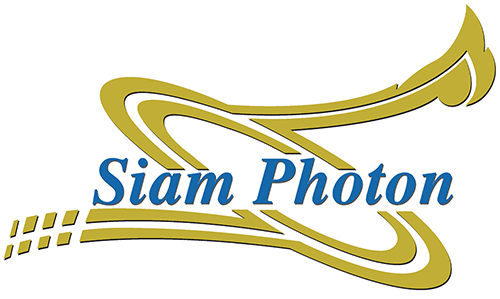The storage ring is to store the electron beam for long period of time, and synchrotron radiation is emitted when electrons are curved in the magnetic field of storage ring.

Major parameters

 Beam energy 1.2 GeV Circumference 81.3 m Stored beam current (nominal) 150.0 mA Lattice DBA (Double Bend Achromat) Superperiod 4 Length of straight section 5.2 m × 4 Max RF voltage,  VRF 200 kV (300kV max.) RF frequency, fRF 118.000 MHz Harmonic number 32 Energy spread 0.066 % Energy loss per turn (Bare ring) 65.94 keV Synchrotron oscillation frequency, fs 13.5 kHz Betatron tune, vx / vy 4.75/2.82

Magnet parametersBending magnet

 Number of bending magnets = 8 Bending radius ( ρ ) = 2.78 m Deflection angle ( ß ) = 45.0 ° ( 0.785398 rad ) Path Length (l) = 2.1834 m Magnetic Field (B) = 1.2 T Magnet type : C-type

 Number of quadrupole families = 4

QF1

 The number of QF1 magnets = 8 Mechanical core length = 0.290 m Effective length = 0.323 m Maximum current = 500 A B/I slope = 0.025738 T/m.A

QD2

 The number of QD2 magnets = 8 Mechanical core length = 0.290 m Effective length = 0.323 m Maximum current = 500 A B/I slope = 0.025745 T/m.A

QF3

 The number of QF3 magnets = 8 Mechanical core length = 0.290 m Effective length = 0.323 m Maximum current = 400 A B/I slope = 0.026045 T/m.A

QD4

 The number of QD4 magnets = 8 Mechanical core length = 0.290 m Effective length = 0.323 m Maximum current = 400 A B/I slope = 0.026546 T/m.A

Sextupole magnet

 Number of sextupole families = 2

SF

 The number of SF magnets = 8 Mechanical core length = 0.15 m Maximum current = 250 A B/I slope = 5.4733 T/m2.A

SD

 The number of SD magnets = 8 Mechanical core length = 0.20 m Maximum current = 250 A B/I slope = 5.4885 T/m2.A

Steering magnet

Horizontal steering magnet

 Number of horizontal steering magnet = 16 Mechanical core length = 0.1 m Effective length = 0.16 m Maximum current = 25 A Pole gap = 0.16 m Magnetic field = 0.080 T Magnet type = H Type Solid

Vertical steering magnet

 Number of Vertical steering magnet = 12 Mechanical core length = 0.1 m Effective length = 0.23 m Maximum current = 25 A Pole gap = 0.161 m Magnetic field = 0.038 T Magnet type = H Type Solid

Injection septum magnet (S-SMI)

 Bending radius ( ρ ) = 2.78 m Deflection angle ( ß ) = 15.0 ° ( 0.2617994 rad ) Path Length (l) = 0.8725774 m Magnetic Field (B) = 1.199 T Maximum current = 5000 - 8050 A Pulse width = 250 µs

Bump magnet(S-BMP1,S-BMP2 and S-BMP3)

 Mechanical core length = 0.295 m Maximum current = 700 – 2915 A

Synchrotron Light Research Institute,111 University Avenue, Muang District, Nakhon Ratchasima 30000, THAILAND
Postal address : P.O. Box 93, Muang, Nakhon Ratchasima 30000, THAILAND

Tel.: 66 44 217 040 Fax: 66 44 217 047 Email: siampl@slri.or.th